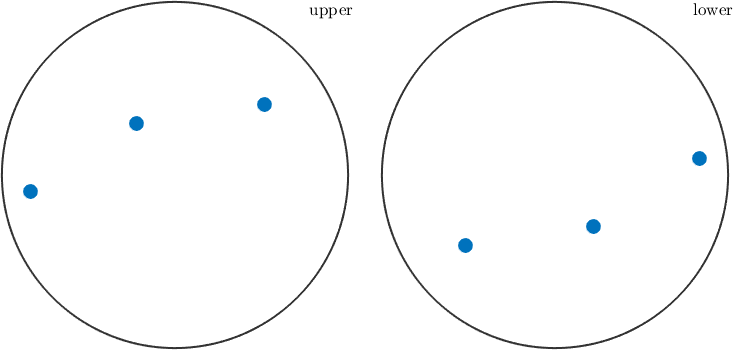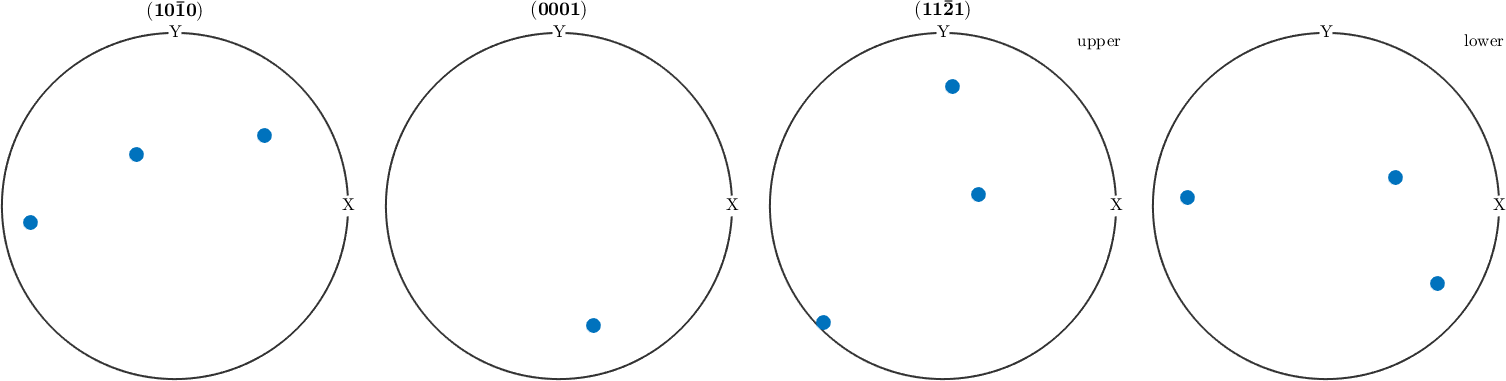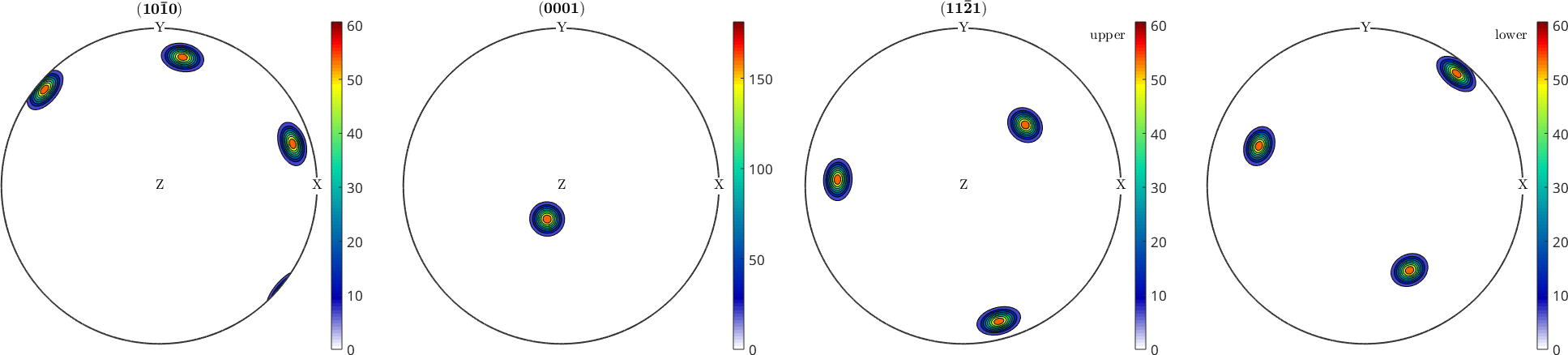Pole Figures edit page

Pole figures are two dimensional representations of orientations. To illustrate this we define a random orientation with trigonal crystal symmetry

Starting point is a fixed crystal direction h, e.g.,

Next the specimen directions corresponding to all crystal directions symmetrically equivalent to h are computed

and ploted in a spherical projectionSince the trigonal symmetry group has six symmetry elements the orientation appears at six possitions.

A shortcut for the above computations is the commandWe observe, that for some crystal directions only the upper hemisphere is plotted while for other upper and lower hemisphere are plotted. The reason is that if h and -h are symmetrically equivalent the upper and lower hemisphere of the pole figure are symmetric as well.

## Contour plots